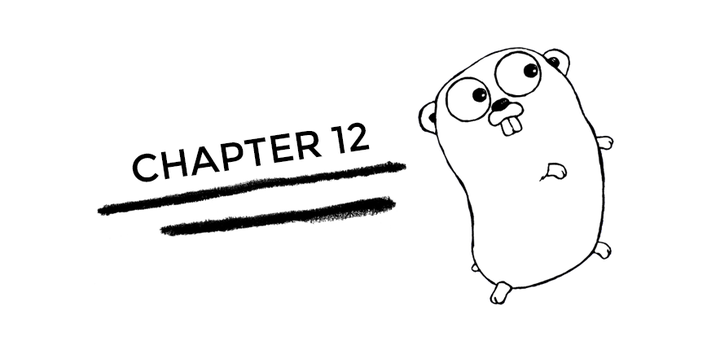# Golang - Chapter 12

MapsMaps (dictionaries, hash maps). Initializing a map can be done literally or via the `make` function.

``````// maps a string to an int
m := make(map[string]int)
n := map[string]int{"foo": 1, "bar": 2}``````

We access the map (with the key) and insert/update values as shown below:

``m["k1"] = 7``

We can remove a key with the `delete` method.

``delete(m, "key1")``

We can get the size of a map using the `len` method, i.e. `len(m)`.

If we access a key that does not exist, Go returns a zeroed value. There are 3 possibilities when we access a map:

• Key exists
• Key does not exists
• Key exists with an zeroed value or Key does not exist and hence there a zeroed value is returned (ambiguous)

To prevent the ambiguity in the 3rd case, we use this syntax: `elem , ok := m[key]`. The returned variable `ok` is a boolean type which indicates if the key was present or not.

There is no order to it, iterating over a map does not guarantee any particular order.

Passing maps to a function passes the pointer of the map. Modifying the map within a function will modify the original map.

## Range Operation

The `range` keyword is used to iterate on slices and maps. The range operation returns two values for every iteration - first is the index and second is the element at that index.

``````nums := []int{1, 2, 3, 4}
sum := 0
// here we have ignored the first return value, the index
for _, num := range nums {
sum += num
}``````
``````kvs := map[string]string{"a": "apple", "b": "banana"}
for k, v := range kvs {
fmt.Printf("%s -> %s\n", k, v)
}``````

Exercise!

Create a map, iterate over it using the range operation and print them out to the console.I code.